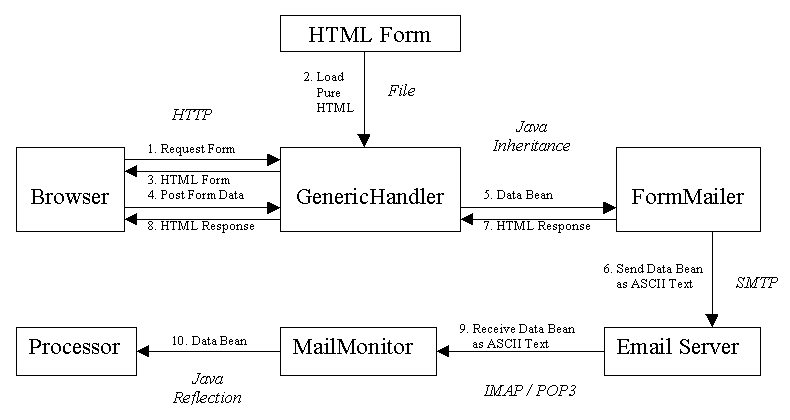# 12. Email-based Application

The next few chapters describe a full application based on Devsphere Mapping Framework and the JavaMail standard extension. It contains a servlet called FormMailer and a standalone application called MailMonitor.

## 12.1. Application Model

The FormMailer servlet uses the mapping framework and its helper packages to convert form data to bean objects and then to key-value pairs. The obtained ASCII text is emailed to one or more addresses using JavaMail.

The MailMonitor application uses JavaMail to get the messages from the e-mail server. Then, it converts the obtained key-value pairs to bean objects, which are forwarded to a dynamically loaded class for processing.The HTML form may look like this:

```<HTML>
<BODY>
<FORM METHOD="POST">
<P> String <BR>
<INPUT TYPE="TEXT" NAME="string" SIZE="20">

<P> Number <BR>
<INPUT TYPE="TEXT" NAME="number" SIZE="20">

<P> Integer <BR>

<P> Flag <BR>
<INPUT TYPE="CHECKBOX" NAME="flag" VALUE="true">Flag

...

<P>
<INPUT TYPE="SUBMIT" VALUE="Submit">
<INPUT TYPE="RESET" VALUE="Reset">
</FORM>
</BODY>
</HTML>```

The HTML form is mapped to a JavaBean like this

```public class SimpleBean implements java.io.Serializable {
private String string;
private float number;
private int integer;
private boolean flag;
...

public String getString() { return this.string; }
public void setString(String value) { this.string = value; }

public float getNumber() { return this.number; }
public void setNumber(float value) { this.number = value; }

public int getInteger() { return this.integer; }
public void setInteger(int value) { this.integer = value; }

public boolean getFlag() { return this.flag; }
public void setFlag(boolean value) { this.flag = value; }
...
}```

The valid bean objects would be processed by a class like this:

```public class SimpleProc {
public void processSimpleBean(SimpleBean simpleBean) {
...
}
}```

## 12.2. Hints and Tips

The following chapters present

• a servlet that may be used to send the form data to one or more email addresses,
• the store connectors, which are components that communicate with a message store,
• the core class of the application, which invokes the connectors' methods and deals with the multithreading issues,
• the class that starts the mail monitor application,
• an example that uses the form mailer and the mail monitor.